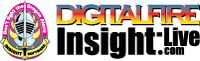•The secret to cool bodies and glazes is a lot of testing.
•The secret to know what to test is material and chemistry knowledge.
•The secret to learning from testing is documentation.
•The place to test, do the chemistry and document is an account at https://insight-live.com
•The place to get the knowledge is https://digitalfire.com

Sign-up at https://insight-live.com today.

# Ball Clay R

 Oxide Analysis Formula CaO 0.10% 0.009 MgO 0.58% 0.071 K2O 2.70% 0.142 Na2O 0.17% 0.014 TiO2 1.32% 0.082 Al2O3 20.60% 1.000 SiO2 67.00% 5.520 Fe2O3 0.93% 0.029 LOI 6.20 Oxide Weight 462.47 Formula Weight 493.03

## XML for Import into INSIGHT

<?xml version="1.0" encoding="UTF-8"?> <material name="Ball Clay R" descrip="" searchkey="" loi="0.00" casnumber=""> <oxides> <oxide symbol="CaO" name="Calcium Oxide, Calcia" status="" percent="0.100" tolerance=""/> <oxide symbol="MgO" name="Magnesium Oxide, Magnesia" status="" percent="0.580" tolerance=""/> <oxide symbol="K2O" name="Potassium Oxide" status="" percent="2.700" tolerance=""/> <oxide symbol="Na2O" name="Sodium Oxide, Soda" status="" percent="0.170" tolerance=""/> <oxide symbol="TiO2" name="Titanium Dioxide, Titania" status="" percent="1.320" tolerance=""/> <oxide symbol="Al2O3" name="Aluminum Oxide, Alumina" status="" percent="20.600" tolerance=""/> <oxide symbol="SiO2" name="Silicon Dioxide, Silica" status="" percent="67.000" tolerance=""/> <oxide symbol="Fe2O3" name="Iron Oxide, Ferric Oxide" status="" percent="0.930" tolerance=""/> </oxides> <volatiles> <volatile symbol="LOI" name="Loss on Ignition" percent="6.200" tolerance=""/> </volatiles> </material>